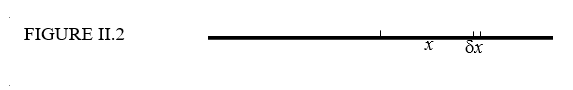$$\require{cancel}$$

2.3: Moments of Inertia of Some Simple Shapes

•• Contributed by Jeremy Tatum
• Emeritus Professor (Physics & Astronomy) at University of Victoria

A student may well ask: "For how many different shapes of body must I commit to memory the formulas for their moments of inertia?" I would be tempted to say: "None". However, if any are to be committed to memory, I would suggest that the list to be memorized should be limited to those few bodies that are likely to be encountered very often (particularly if they can be used to determine quickly the moments of inertia of other bodies) and for which it is easier to remember the formulas than to derive them. With that in mind I would recommend learning no more than five. In the following, each body is supposed to be of mass $$m$$ and rotational inertia $$I$$.

Formula 1.

A rod of length $$2l$$ about an axis through the middle, and at right angles to the rod:

$I = \dfrac{1}{3}ml^{2} \tag{2.3.1}\label{eq:2.3.1}$

Formula 2.

A uniform circular disc of radius $$a$$ about an axis through the center and perpendicular to the plane of the disc:

$I = \dfrac{1}{2}ma^{2} \tag{2.3.2}\label{eq:2.3.2}$

Formula 3.

A uniform right-angled triangular lamina about one of its shorter sides - i.e. not the hypotenuse. The other not-hypotenuse side is of length $$a$$:

$I = \dfrac{1}{6}ma^{2} \tag{2.3.3}\label{eq:2.3.3}$

Formula 4.

A uniform solid sphere of radius $$a$$ about an axis through the center.

$I = \dfrac{2}{5}ma^{2} \tag{2.3.4}\label{eq:2.3.4}$

Formula 5.

A uniform spherical shell of radius $$a$$ about an axis through the center.

$I = \dfrac{2}{3}ma^{2} \tag{2.3.5}\label{eq:2.3.5}$

I shall now derive the first three of these by calculus. The derivations for the spheres will be left until later.

1.Rod, length $$2l$$ (Figure II.2)The mass of an element $$\delta x$$ at a distance $$x$$ from the middle of the rod is $$\dfrac{m\delta x}{2l}$$.

and its second moment of inertia is

$\dfrac{mx^{2}\delta x}{2l}.$

The moment of inertia of the entire rod is

$\dfrac{m}{2l} \int_{-l}^{l} x^{2} dx = \dfrac{m}{l} \int_0^l x^{2} dx = \dfrac{1}{3}ml^{2}.$

2. Disc, radius $$a$$ . (Figure II.3)The area of an elemental annulus, radii $$r$$ is $$r + \delta r$$ is $$2 \pi r \delta r$$.

The area of the entire disc is $$\pi a^{2}$$.

Therefore the mass of the annulus is

$\dfrac{2 \pi r \delta rm}{\pi a^{2}} = \dfrac{2mr\delta r}{a^{2} } .$

and its second moment of inertia is

$\dfrac{2mr^{3}\delta r}{a^{2}}.$

The moment of inertia of the entire disc is

$\dfrac{2m}{a^{2}} \int_{0}^{a} r^{3} dr = \dfrac{1}{2}ma^{2}.$

3. Right-angled triangular lamina. (Figure II.4)The equation to the hypotenuse is $$y = b(1 - x/a)$$.

The area of the elemental strip is $$y \delta x = b(1 - x/a)\delta x$$ and the area of the entire triangle is $$\dfrac{ab}{2}$$.

Therefore the mass of the elemental strip is $$\dfrac{2m(a - x)\delta x}{a^{2}}$$.

and its second moment of inertia is $$\dfrac{2mx^{2}(a - x)\delta x}{a^{2}}$$.

The second moment of inertia of the entire triangle is the integral of this from $$x = 0$$ to $$x = a$$ , which is $$\dfrac{ma^{2}}{6}$$.

Uniform circular lamina about a diameter.

For the sake of one more bit of integration practice, we shall now use the same argument to show that the moment of inertia of a uniform circular disc about a diameter is $$\dfrac{ma^{2}}{4}$$. However, we shall see later that it is not necessary to resort to integral calculus to arrive at this result, nor is it necessary to commit the result to memory. In a little while it will become immediately apparent and patently obvious, with no calculation, that the moment of inertia must be $$\dfrac{ma^{2}}{4}$$. However, for the time being, let us have some more calculus practice. See Figure II.5.The disc is of radius $$a$$, and the area of the elemental strip is $$2y \delta x$$. But $$y$$ and $$x$$ are related through the equation to the circle, which is $$y=(a^{2}-x^{2})^{1/2}$$. Therefore the area of the strip is $$2(a^{2} - x^{x})^{1/2} \delta x$$. The area of the whole disc is $$\pi a^{2}$$, so the mass of the strip is

$m \times \dfrac{2(a^{2} - x^{2})^{1/2}\delta x}{\pi a^{2}} = \dfrac{2m}{\pi a^{2}} \times (a^{2} - x^{2})^{1/2}\delta x.$

The second moment of inertia about the $$y$$-axis is

$\dfrac{2m}{\pi a^{2}} \times x^{2}(a^{2} - x^{2})^{1/2}\delta x.$

For the entire disc, we integrate from $$x = -a$$ to $$x = +a$$ , or, if you prefer, from $$x = 0$$ to $$x = a$$ and then double it. The result $$\dfrac{ma^{2}}{4}$$ should follow. If you need a hint about how to do the integration, let $$x = a cos \theta$$ (which it is, anyway), and be sure to get the limits of integration with respect to $$\theta$$ right.

The moment of inertia of a uniform semicircular lamina of mass $$m$$ and radius $$a$$ about its base, or diameter, is also $$\dfrac{ma^{2}}{4}$$, since the mass distribution with respect to rotation about the diameter is the same. $$\dfrac{ma^{2}}{4}$$, since the mass distribution with respect to rotation about the diameter is the same.